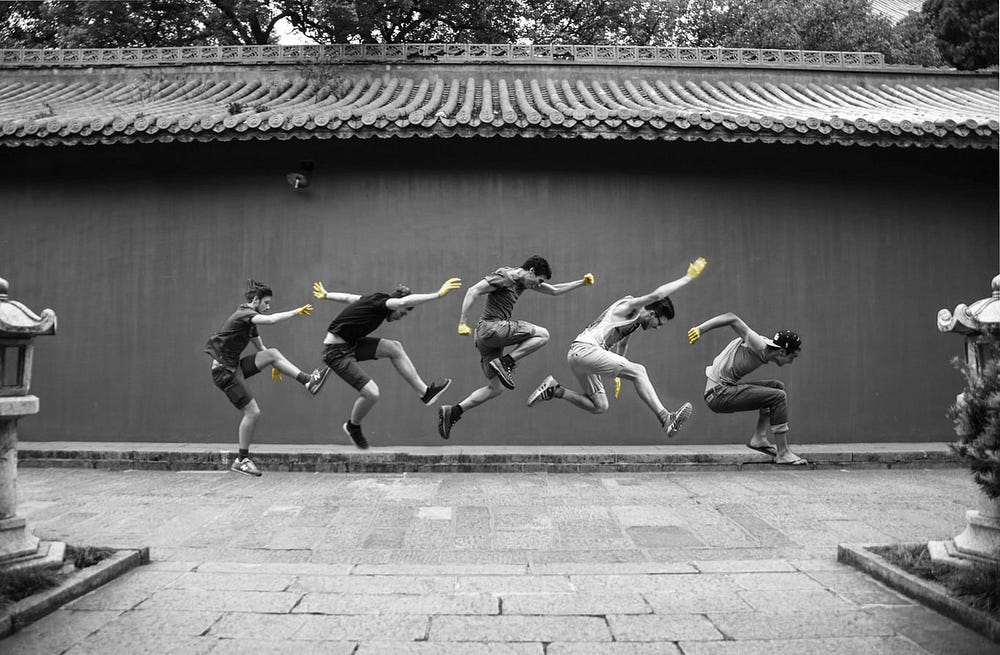# HAMR — 3D Hand Shape and Pose Estimation from a Single RGB Image

Source: Deep Learning on Medium## 2.1. 2D Pose Estimation

Similar to other recent methods, the authors employ a cascade encoder-decoder network to predict the 2D Gaussian like heat-maps for 2D pose estimation, which is noted as Φ in Equation 1 below.

Here, K indicates the number of joints and {H, W} are the resolutions of the heat-maps. Each keypoint has a corresponding heat-map, and each pixel value on the heat-map indicates the confidence of the keypoint located in that given 2D position.

In addition, as perspective ambiguity cannot be resolved when applying direct regression from 3D pose heat-maps, the authors concatenate the intermediate-layer features with the M heat-maps, and then feed them into the following iterative regression module for additional information.

## 2.2. Hand Mesh Recovery

2.2.1 Hand Mesh Representation

MANO (hand Model with Articulated and Non-rigid defOrmations) is utilized as the generic 3D hand model in the HAMR framework. In particular, MANO factors hand mesh into its shape — it mainly models hand properties such as finger slenderness and palm thickness and pose.

MANO parameterizes a triangulated mesh M ∈ R^{N×3} with a set of parameters θ_mesh = {β , θ }, where β ∈ R¹⁰ denotes the shape parameters and θ ∈ R^{K×3} denotes the pose parameters. Technically speaking, β represents the coefficients of PCA components that sculpt the identity subject, and θ denotes the relative 3D rotation of K joints on a Rodrigues Vector representation.